# 1st PUC Chemistry Question Bank Chapter 10 The S-Block Elements

## Karnataka 1st PUC Chemistry Question Bank Chapter 10 The S-Block Elements

### 1st PUC Chemistry The S-Block Elements One Mark Questions and Answers

Question 1.
How does the density of alkali metal change from Li to Cs?
Density increases down the group from Li to Cs.

Question 2.
Elements of which group in the periodic table belong to s-block?
I and II groups.

Question 3.
In what way the electronic configuration of hydrogen is similar to that of the electronic configuration of alkali metals?
Hydrogen and alkali metals both have one electron in the outermost orbital.

Question 4.
What similarity is found in the electronic configurations of hydrogen and halogen?
Both hydrogen and halogen are in short of one electron for the completion of the outermost orbital containing electrons.

Question 5.
Name any four alkali metals.
Lithium, Sodium, Potassium and Rubidium.

Question 6.
LiCI and MgCk dissolve in alcohol. How do you explain this?
Both LiCI and MgCl2 are covalent compounds and dissolve in alcohol. This is due to high polarizing power of Li+ and Mg2+ ions.Question 7.
The alkali metals have no tendency to show variable oxidation states. Give reason.
Alkali metals show oxidation state of +1. With the loss of valence electron it attains the stable configuration of nearest inert gas. Its second ionization potential is high. Hence an alkali metal does not show variable oxidation states.

Question 8.
Write the alkali metals in the increasing order of hydration energy.
Li + > Ma+ > K+> > Rb+ > Cs+

Question 9.
Why are group 1 elements called alkali metals ?
It is because their hydroxides are soluble bases called alkalies. Secondly their ashes are alkaline in nature.

Question 10.
Why do alkali metals have low ionisation energy ?
It is due to largest atomic size, they can lose electrons easily.

Question 11.
Alkali and alkaline earth metals cannot be obtained by chemical reduction, why?
Alkali and alkaline metals are good reducing agents, they cannot be obtained by chemical reduction.

Question 12.
Why does ionisation energy of alkali metals decrease with the increase in atomic number ?
Atomic size increases with increase in atomic number, therefore, nuclear force of attraction between valence electrons and nucleus decreases, hence ionisation energy decreases down the group.

Question 13.
Why group 2 elements (Mg and Ca) are harder and denser than group 1 elements ?
They have strong metallic bonds due to smaller size and have more number of valence electrons.

Question 14.
Why is potassium more reactive than sodium ?
K has lower ionisation energy than sodium due to bigger atomic size, therefore, it is more reactive.

Question 15.
Why are alkali metals strong reducing agents?
It is because of low ionisation energy. They can lose electrons easily, that is why they are strong reducing agents.

Question 16.
Why are alkali metals used in photoelectric cells ?
They have low ionisation energy and can lose electrons when light falls on them, that is why they are used in photoelectric cells.

Question 17.
Write electronic configuration of Na (11) and K (19).
Na(11): 1s22s22p63s1 K(19): ls22s22p63s13p64s1

Question 18.
Why do alkali metals have low melting and boiling points ?
It is due to weak metallic bonds which is due to bigger atomic size that is why they how low melting and boiling points.

Question 19.
How will you prepare sodium hydrogen carbonate from sodium chloride ?(NH3)HCO3 + NaCl → NaHCO3 + NH4Cl

Question 20.
Why do alkali metals not occur in free state ?
They are highly reactive, therefore, they occur in combined state and do not occur in free state.Question 21.
Why is second ionisation energy of alkali metals higher than alkaline earth metals ?
Alkali metals acquire, noble gas configuration after losing 1 electron, therefore their second ionization energy is higher than alkaline earth metals.

Question 22.
Which out of K, Mg, Ca and Al from amphoteric oxide ?
Al forms amphoteric oxide, i.e., acidic as well as basic in nature.

Question 23.
Which out of Na, K, Al, Mg occur as oxide in nature ?
Al occurs as oxide in nature as bauxite Al2O3. 2H2O

Question 24.
Why do alkali metals give characteristic flame colouration?
They have low ionization energy and absorb energy from visible region of spectrum and radiate complementary colour.

Question 25.
What happens when K burns in air ? Give chemical equation.
K + O2 → KO2, pottassium superoxide

Question 26.
What is quick lime ? How is it prepared ?
Quick lime is calcium oxide. It is prepared by heating limestone.

Question 27.
Give two uses of plaster of paris. Also give its formula.

1. It is used in plastering fracture bones,
2. It is used in preparations of chalks. Its formula is (CaSO4)2.H2O

Question 28.
Arrange the following in order of their increasing covalent character : MCI, MBr, MF, Ml (Where M is alkali metal)
MF < MCI < Ml, lesser the difference in electronegativity, more will be covalent character.

Question 29.
One reason on being heated in excess supply of air K, Rb and Cs from superoxide in preference to oxides and peroxides ?
K, Rb and Cs are more reactive therefore, they form superoxide in preference to oxides and peroxides K+,Rb+ and Cs+ ions are large cations and superoxide ion $$\mathrm{O}_{2}^{-}$$, is also large. Larger cations stabilize larger anions, therefore, they form superoxide.

Question 30.
What happens when KO2 reacts with water ? Give balanced chemical equation.
2KO2 (Pottasium sup eroxide) + 2H2O → 2KOH + O2 + H2O2

Question 31.
Complete the reaction : Lil + KF →
Lil + KF → LiF + KI; larger cation stabilizes larger anion and smaller cation stabilizes smaller anion.

Question 32.
Name the reagent or one process to distinguish between :

• BeSO4 and BaSO4
• Be(OH2) and Ba(OH)2

• BeSO4 is soluble in water while BaSO4 is not.
• Be(OH)2 dissolves in NaOH while Ba(OH)2 is insoluble.

Question 33.
Why does Be resemble Al?
Be resembles Al because charge over radius ratio is similar, i.e., they have similar polarizing power.

Question 34.
The second ionization enthalpy of Ca is higher than first and yet calcium forms CaCl2 and not CalCl Why?
The hydration energy of Ca2+ over comes the second ionization energy of Ca, that is why Ca forms CaCl2 and not CaCl. Ca+ is not stable

Question 35.
Name the alkali metals which form superoxide when heated in air?
K, Rb, Cs are alkali metals which form superoxide when heated in air.Question 36.
Name the metal which floats on water without apparent reaction.
Berylium.

Question 37.
Why is BeCl2 soluble in organic solvents ?
BeCl2 is covalent, therefore, soluble in organic solvents.

Question 38.
Starting from quick lime how slaked lime is prepared ? Is this reaction exothermic or endothermic ?
CaO + H2O → Ca(OH)2 +heat
When CaO is put in water, it forms calcium hydroxide. It is an exothermic reaction.

Question 39.
Carbon dioxide is passed through a suspension of limestone in water. Write balanced chemical equation for the above reaction.
CaCO3 + H2O + CO2 → Ca(HCO3)2

Question 40.
What do we get when crystals of washing soda exposed to air?
We get amorphous sodium carbonate because it loses water molecules.

Question 41.
What happens when sodium dissolve in liquid ammonia?
It results in the formation of intense blue colour solution which possess conducting power
$$\mathrm{Na}+(\mathrm{x}+\mathrm{y}) \mathrm{NH}_{3} \longrightarrow \mathrm{Na}\left(\mathrm{NH}_{3}\right)_{\mathrm{x}}^{+}+\left[\mathrm{e}\left(\mathrm{NH}_{3}\right)_{3}\right]^{-}$$

Question 42.
Name the elements (alkali metals) which form superoxide when heated in excess of air.
Potassium, rubidium and caesium

Question 43.
Why is oxidation state of Na and K always + 1?
It is due to their high second ionization enthalpy and stability of their ions [Na+ K+]

Question 44.
What is meant by dead burnt plaster?
It is anhydrous calcium suplhate (CasO4)

Question 45.
What is the reason that sodium reacts with water more vigorously than lithium ?
Because sodium is more electro-positive than Li.

Question 46.
Why is sodium thiosulphate used in photography ?
Because of its complex forming behaviour.

Question 47.
Why does lithium show anomalous behaviour ?
Due to its small size and high charge/size ratio.### 1st PUC Chemistry The S-Block Elements Two Marks Questions and Answers

Question 1.
Give reason for diagonal relationship of lithium with magnesium.
Both Lithium and magnesium have small size and high charge density. The electronegativities of Li is 1.0 and Mg is 1.2. They are low and almost same. Their ionic radii are similar. Hence they show similarities which is known as diagonal relationship between first element of a group with the second element in the next higher group.

Question 2.
What is photoelectric effect?
Alkali metals have the lowest ionization energy in each period of the periodic table. Hence they emit electrons even when exposed to light. This phenomenon is called photoelectric effect. Rubidium and caesium are used in photoelectric cells.

Question 3.
Give two important ores each of Na and K.
Rock salt (NaCl), Na2CO3, NaHCO3, 2H2O (trona) are important ores of Na. Sylvine (kCl); kCl.mgcl2.6H2O (carnallite) are of k.

Question 4.
Give one important use of following compounds.

1. NaHCO3
2. Slaked lime
3. NaOH

1. Sodium bicarbonate is used as antacid
2. Slaked lime is used for white washing,
3. NaOH is used in manufacture of soap.

Question 5.
What is effect of heat on the following compounds ? (Give equations for the reactions)
(i) CaCO3
(ii) CaSO42H2OQuestion 6.
Name the metals which are found in each of the following minerals :
(a) Chile salt petre
(b) Marble
(c) Epsomite
(d) Bauxite
(a) Na
(b) Ca
(c) Mg
(d) Al.

Question 7.
What are the raw materials used in manufacture of Portland cement ? How is it manufactured ?
Limestone and clay are raw materials used in manufacture of cement. It is prepared by heating powdery mixture of limestone and clay in dry process. In wet process, fine – powdered mixture is converted into slurry by adding water and then it is heated at a temperature 1500° C to 1600° C, the product formed is called clinkers. It is cooled down and mixed with gypsum (CaSO4, 2H2O) and then it is powdered.

Question 8.
What is composition of Portland Cement ? What is average composition of good quality cement ?
CaO = 50% ; 60% ; SiO2 = 20% to 25% ; Al2O3 = 5 to 10% ; MgO = 2% to 3% ; Fe2O = 1 to .2% ; SO2 = 1 to 2% is composition of Portland cement.
The ratio of SiO2 (silica) to alumina (Al2O3) should be between 2.5 and 4.0 and the ratio of lime (CaO) to total oxides of silicon, aluminium and iron (SiO2, Al2O3 and Fe2O3) should be as close to 2 as possible.

Question 9.
Describe in brief the manufacture of caustic soda using the Castner-Kellner cell.
The Castner-Kellner cell consists of large rectangular trough divided into three compartments with partition short of reaching the bottom of the tank. Thus mercury in one compartment can flow into another but solution cannot mix. Graphite anodes are used in outer compartments filled with NaCl solution. The middle compartment contains very dilute solution of caustic soda and filled with iron rods as cathode.

On passing electric current CI2 is liberated in outer compartments and sodium liberated at cathode. Mercury forms amalgam which is passed into middle compartment in which mercury acts as anode (having induced +ve potential).
At anode Na+ + e → Na
At cathode Na + Hg → Na – Hg
2(Na – Hg) + 2H2O → 2NaOH + Hg + H2
The concentration of NaOH goes on increasing in the middle compartment. When the concentration of NaOH reaches 20% the solution is replaced by dilute solution.

Question 10.
Compare four properties of alkali metals and alkaline earth metals.

 Alkali Metals Alkaline earth metals 1. The show + 1 oxidation state. 1. They show + 2 oxidation state. 2. They are soft metals 2. They are harder than alkali metals. 3. They do not form complexes except Li. 3. They can form complex compounds 4. Their carbonates are soluble in water except Li2CO3 4. Their carbonates are insoluble water.Question 11.
What happens when exhaling is made through a tube passing in lime water ? What will happen if continued exhaling is made through it ? If the solution thus obtained is heated, what do we observe ? Explain giving chemical reactions.
Lime water turns milky due to formation of CaCO3
Ca(OH)2 + CO2 → CaCO3 + H2O
Milkiness will disappear if continuously exhaling i.e., CO2 is passed due to formation of calcium bicarbonate.
CaCO3 + CO2 + H2O → Ca(HCO3)2
Milkiness will reappear if heating is done due to formation. CaCO3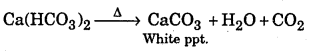Question 12.
Complete the following equations :
(i) Ca + N2
(ii) Ca + SO2
(iii) Ca(OH)2+NH4Cl →
(iv) Ca + CO2
(i) 3 Ca + N2 → Ca3N2
(ii) 2 Ca + SO2 → 2CaO + S
(iii)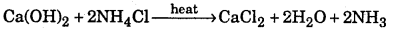(iv) 2 Ca + CO2 → CaO + C

Question 13.
What is dead burnt plaster ? How is it obtained from gypsum?
CaSO4 is called dead burnt plaster. It is obtained by heating gypsum at high , temperature.

Question 14.
What is used for drying alcohol and non-acidic gases and why ?
Calcium is used for drying alcohol and non-acidic gases because Ca does not react with alcohol.

Question 15.
What is the mixture of CaCN2 and carbon called ? How is it prepared ? Give its uses.
It is called Nitrolim. It is prepared by heating CaC2 with N2 at high temperature. It is used as fertilizer.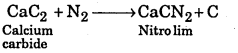Question 16.
Convert limestone to calcium carbide.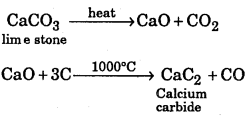Question 17.
What are isomorphous salts ? Give two examples.
Isomorphous salts are those which have same crystalline structure, e.g., MgSO4, 7H2O and ZnSO4,7H2O are isomorphous.

Question 18.
Which metal is present in chlrophyll ? How does this metal react with N2 ?
Mg is present in chlorophyll. N2 reacts with Mg to form magnesium nitride.
3Mg + N2 → Mg,N2 (magnesium nitride)

Question 19.
Name an alkali metal carbonate which is thermally unstable and why ? Give its decomposition reaction.
Li2CO3 is thermally unstable because it is covalent. It decomposes to form Li2O and
CO2 ; Li2CO3 → Li2O + CO2

Question 20.
Why are ionic hydrides of only alkali metals and alkaline earth metals are known ? Give two examples.
Alkali metals and alkaline earth metals aye most electropositive due to low ionization energy or enthalpy. Therefore, they can form ionic hydrides, e.g., NaH, KH and CaH2.

Question 21.
Which one of the alkaline earth metal carbonate is thermally most and last stable. Why ?
BaCO3 is thermally most satable due to greater ionic character and high lattice energy whereas BaCO3 is thermally least stable because it is covalent and has less lattice energy.

Question 22.
Which out of Li, Na, K, Be, Mg, Ca has lowest ionization enthalpy and why ?
K has lowest ionisation energy due to larger atomic size among these elements. The force of attraction between valence electron and nucleus is less, therefore, it can lose electron easily.

Question 23.
Which alkali metal ion forms largest hydrate ion in aqueous solution and why?
Li+ forms largest hydrated cations because it has highest hydration energy. It has smallest size therefore, it is most hydrated.

Question 24.
What is responsible for the blue colour of the solution of alkali metal in liquid ammonia ? Give chemical equation also. [MSE (Chandigarh) 2003]
The solvated electron, [e(NH3)] or ammoniated electron is responsible for blue colour of alkali metal solution in NH3. It absorbs light from visible region and radiates complementary colour, (in the equation am = ammoniated)
Na+(am) + e(am) + NH2(I) →NaNH2(am) + $$\frac { 1 }{ 2 }$$H2(g)

Question 25.
Heat of Hydration of Na+ (size 102 pm) = -397 kJ mol-1 whereas Caz 100 pm) = -1650 kJ mol-1. Explain the difference.
Ca2+ is smaller in size than Na+ and also it has higher charge, therefore, its hydration energy is more than that of Na+.Question 26.
Discuss the diagonal relationship of Be and A1 with regard to
1. action of alkali and
2. the structure of their chloride.
1. Be and A1 both react with NaOH to form sodium beryllate and sodium meta aluminate respetively. Be dissolves in excess of NaOH to form [Be(OH)4]2 where as
Al forms [ A1 (OH)6 ]3- in excess of NaOH.
2H2O + Be + 2NaOH → Na2 [Be (OH)4 ] (saliun beryllali) + H2
2A1 + 6NaOH + 6H2O → 2Na3 [ A1 (OH)6 ] (Sodiam meta ilumivali) + 3H2

2. BeCl2 is electron deficient, therefore, has polymeric chain structure in solid state.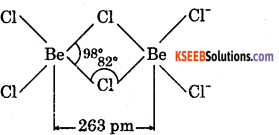AlCl3 is also electron deficient, It exists as dimmer, i.e., Al2Cl6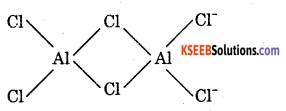Question 27.
Complete the following:
(i) Li + N2
(ii) $$\text { LiNO }_{3} \stackrel{\text { heat }}{\longrightarrow}$$
(iii) $$\mathrm{NaNO}_{3} \stackrel{\text { heat }}{\longrightarrow}$$
(iv) B2H6Question 28.
Arrange the (i) hydroxide and (ii) sulphates of alkaline earth metals in order of decreasing solubilities giving a suitable reason for each.
Ba(OH)2 > Sr(OH)2 > Ca(OH)2 > Mg(OH)2 > Be(OH)2
Solubility of hydroxides goes on increasing down the group because hydration energy dominates over lattice energy.
BaSO4 < SrSO4 < CaSO4 < MgSO4 < BeSO4

Question 29.
What makes lithium show properties uncommon to the rest of alkali metals ? Write two points of similarly in properties between lithium and magnesium.
Solubility of sulphates goes on decreasing down the group because lattice energy dominates over hydration energy. Lithium has smallest atomic size and highest ionization energy, highest polarizing power that is why it shows uncommon properties to the rest of alkali metals.

• Both Lithium and Magnesium react with N2 to form nitrides.
• Li and Mg react with O2 to form monoxides.

Question 30.
Write the chemical equations of the reactions involved in solvay process of preparation of sodium carbonate.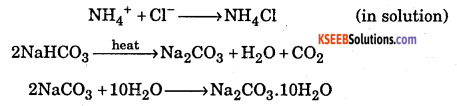Question 31.
Arrange the following in order of the increasing covalent character : MCI, MBr, MF, MI (where M = alkali metal)
As the size the anion increases, covalent character increases and hence the order is ‘MF < MCI < MBr < MI.

Question 32.
What is the formula of gypsum? What happens when it is heated?
CaSO4. 2H2O. When heated to 393 K, it gives plaster of paris (CaSO4.1/2H2O ) but at 473 K it gives dead burnt plaster (CaSO4).

Question 33.
The E° for C1/Cl2 is 1.36, for I/I2 is +0.53, for Ag+/Ag is + 0.79, Na+ is -2.71 and for Li+ / Li is -3.04 V Arrange the following species in decreasing order of reducing strength. I, Ag, Cl Li, Na
The more negative or less positive is the electrode potential, more is the reducing strength of the species. Since the electrode potentials inceases in the order : Li(-3.04V) < Na(-2.7V) < I(0.53 V) < Ag(+0.79V) < Cl (+1.36V), therefore, the reducing strength decreases in the order Li > Na > I > Ag > Cl

Question 34.
How do you prepare KO3 ? Mention Magnetic Behaviour of $$\mathrm{O}_{3}^{-}$$ .
Potassium ozonide (KO3) is formed when ozone is passed through KOH.
2KOH + 5O3 → 2KO3 + 5O2 + H2O
It is an orange coloured solid and contains the paramagnetic O3 ion.

Question 35.
What are ionic polyhide compounds ?
The alkali metals react with halogens and interhalogen compounds forming ionic polyhide compounds.
KI + I2 → K[I3] ; KBr + ICI → K[BrICI] ; KF + BrF3 → K[BrF4]### 1st PUC Chemistry The S-Block Elements Three / Four Marks Questions and Answers

Question 1.
What are alkali metals? Describe their general properties.
1st group elements of periodic table i.e., lithium, sodium, potassium, rubidium and caesium are called alkali metals.
General properties:

• Alkali metals have general electronic configuration ns1E
• Alkali metals exhibit on oxidation state of+1.
• Atomic radius increases down the group from lithium to caesium.
• The metallic property of alkali metals increases from lithium to caesium.
• Alkali metals have low ionization potential.
• Due to low ionization power they are highly electropositive.
• Alkali metals are light metals.

Question 2.
Write the balanced equations from the reaction between
(a) Na2 O2 and water
(b) KO2 and water
(c ) Na2O and CO2
(a) 2Na2O2 + 2H2O → 4NaOH + O2
(b) 2KO2 + 2H2O → 2KOH + H2O2 + O2
(c) Na2O + CO2 → Na2CO3

Question 3.
What is the action of heat on the following compound ?
(i) Na2CO3 and CaCO3 ,
(ii) MgCl2.6H2O and CaCl2.6H2O
(iii) Ca(NO3)2 and NaNO2
(i) Na2CO3 and CaCO3
Na2CO3 does not decompose on heating while CaCO3 evolves CO2
$$\begin{array}{l}{\mathrm{Na}_{2} \mathrm{CO}_{3} \stackrel{\text { heat }}{\longrightarrow} \text { Noaction }} \\ {\mathrm{CaCO}_{3} \stackrel{\text { heat }}{\longrightarrow} \mathrm{CaO}+\mathrm{CO}_{2}}\end{array}$$

(ii) MgCl2.6H2O and CaCl2.6H2O
On heating hydrated CaCl2 is dehydrated while hydrated MgCl2 changes into MgO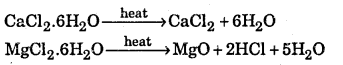(iii) On heating the two nitrate form different products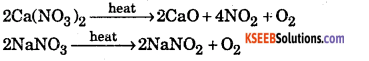Question 4.
Complete the following

1. Ca + H20 →
2. Ca(OH)2 + Cl2
3. BeO + NaOH →

1. Ca + H2O → CaO + H2O
2. Ca(OH)2 + Cl2 → CaOCl2 + H2O
3. BeO + NaOH → Be(OH)2 + Na2O

Question 5.
Explain what happens when
(i) Sodium hydrogen carbonate is heated
(ii) Sodium with mercury reacts with water
(iii) Fused sodium metal reacts with ammonia.Question 6.
Why is it that the s-block elements never occur in free state/nature? What are their usual modes of occurrence and how are they generally prepared?
The elements belonging to s-block in periodic table (i.e. alkali and alkaline earth metals) are highly reactive because of their low ionization energy. They are highly electropositive forming positive ions. So they are never found in free state.
They are widely distributed in nature in the combined state. They occur in earth’s crust in the form of oxides, chlorides, silicates and carbonates.
Generally group I metals are prepared by the electrolysis of fused solution.
As for example: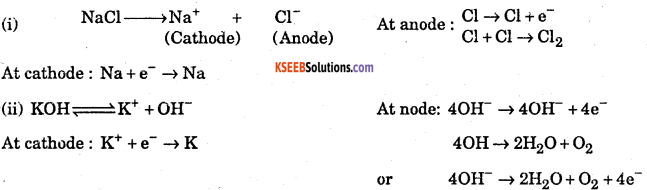These metals are highly reactive and therefore cannot be extracted by the usual methods, because they are strong reducing agents.Question 7.
What is the effect of heat on the following compounds ?
(a) Magnesium chloride hexahydrate
(b) Gypsum
(c) Magnesium sulphate heptahydrate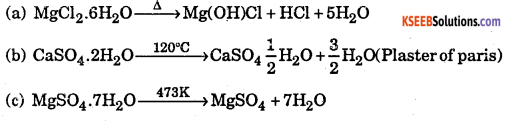Question 8.
What is the approximate composition of Portland cement ? What raw materials are used in the manufacture of this cement ? Describe method.
Raw materials : The raw materials required for the manufacture of cement are lime stone, stone and clay. Lime stone in calcium carbonate, (CaCO3) and it provides calcium oxide. (CaO) Clay is hydrated aluminium silicate, (Al2O3.2SiO2.2H2O) and it provides alumina as well as silica. A small amount of gypsum, CaSO4.2H2O is also required. It is added in calculated quantity in order to adjust the rate of setting of cement.

Manufacture : Cement is made by strongly heating a mixture of lime stone and clay in a rotatory kiln. Lime stone and clay are finely powdered and a little water is added to get a thick paste called slurry. The slurry is fed into a rotatory kiln from the top through the hopper.

The hot gases produce a temperature of about 1770-1870 K in the kiln. At this high temperature at the lime stone and clay present in slimy combine to form cement in the form of small pieces called clinker. This clinker is mixed with 2-3% by weight of gypsum (CaSO4.2 H2O) to regulate the setting time and is then ground to an exceedingly fine powder.
$$\text { Limestone }+\text { Clay } \frac{170-1870 \mathrm{K}}{\text { (Clinker) }} \text { , Cement }+\mathrm{CO}_{2} \uparrow+\mathrm{H}_{2} \mathrm{O} \uparrow$$
When mixed with water the cement reacts to form gelatinous mass which sets to a hard mass when three .dimensional cross lines are formed between silica oxygen silica and silica oxygen aluminium as .
…….. Si – O – Si …….. and Si – O – Al ……….. chains
Composition of cement:
CaO = 50 -60%
SiO2 = 20 – 25%
Al2O3 = 5 – 10%
MgO = 2 – 3%
Fe2G3 = 1-2%
SO3 = 1 – 2%
For a good quality cement the ratio of’silica (SiO2) and alumina (AI2O3) should be between 2.5 to 4.0. Similarly the ratio of lime (CaO) to the total oxide mixtures consisting of SiO2, Al2O3 and Fe2O3 should be roughly 2:1:1, If lime is in excess, the cement cracks during setting. On the other hand, if lime is less than the required, the cement is weak in strength. Therefore, a proper composition of cement must be maintained to get cement of good quality.

Question 9.
Identify A and B in the following reaction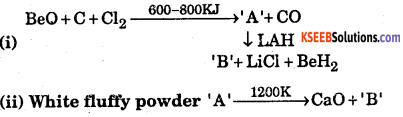(i) ‘A’ is BeCh and ‘B’ is AlCl3
(ii) A is CaCO3, B is CO2

Question 10.
A white crystalline solid ‘A’ on heating loses the water of crystallization to form a monohydrate ‘B’ above 373K, the monohydrate also becomes completely anhydrous and changes to white powder called soda ash. Identify ‘A’ and ‘B’. Also give two uses of ‘A’
‘A’ is Na2CO3.10H2O (Washing soda)
‘B’ is Na2CO3 (Anhydrous sodium carbonate)Uses of‘A’
(a) Used for softening hard water.
(b) Used in glass and soap industries.

Question 11.
Write the uses, and any two reaction of KO2.
Potassium superoxide (KO2) is used as a source of oxygen in submarines, space shuttles and in emergency breathing apparatus such as oxygen masks. Such masks are used in rescue work in mines and in other areas where the air is so deficient in oxygen that an artificial atmosphere must be generated.

The moisture of the breath reacts with superoxide to liberate oxygen, and at that same time the potassium hydroxide formed removes carbon dioxides as it is exhaled thereby allowing the atmosphere in the mask to be continuously regenerated.
4KO2(s) + 2H2O(g) → 4KOH(aq) + 32(g) → KHCO3 (s)
KO2 also combines directly with CO2 forming K2CO3 and with CO2 & moisture forming KHCO3
4KO2 + 2CO2 → 2K2CO3 + 3O2; 4KO2 + 4CO2 + 2H2O → 4KHCO3 + 3O2

Question 12.
(a) Write any four uses of Calcium Hydroxide.
(b) Give chemical equation of the reaction of caustic soda with
1. ammonium chloride, and
2. carbon dioxide
(a)

• It is used in the building material in the form of mortar.
• It is used in the manufacture of bleaching powder.
• It is used in glass making and the purification of sugar.
• It is used to absorbed acidic gases.

(b)
1. NH4Cl + NaOH → NaCl + NH4OH
2. 2NaOH + CO2 → Na2CO3 + H2OQuestion 13.
What is plaster of paris ? How is it prepared ? Give its any two important uses.
Plaster of paris is CaSO4 $$\frac { 1 }{ 2 }$$ H2O. It is prepared by heating gypsum at 373KUses:

• It is used for manufacture of statues
• It is used for filling gaps before white washing

Question 14.
Discuss the trends of:
1. Thermal stability of alkaline earth metal carbonates
2. Solubility of sulphates of group 2 elements
3. Basic strength of alkaline earth metal hydroxides
1. Thermal stability of alkaline earth metal carbonates increases down the group due to increase in ionic character and therefore, increase in lattice energy

2. Solubility of sulphates of group 2 elements decreases down the group because lattice energy dominates over hydration energy.

3. Basic strength of alkaline earth metal hydroxides increases down the group because ionization energy of metal decreases and electropositive character increases down the group.

Question 15.
Explain the different oxides of metals or classify different metal oxides.
Classification of oxides on the basis of oxygen content.
On the basis of oxygen content, oxides can be classified into the following types
1. Normal oxides: Those oxides inwhich the oxidation number of the element (M) can be deducted from the empirical formula MxOy by taking the oxidation number of oxygen as – 2 are called normal oxides. For example, H2O, Na2O, MgO AI2O3, CO2 etc. All these oxides contain M – O bonds.

2. Polyoxides: These oxides contain more oxygen than would be expected from the oxidation number of the element (M). These have have been further classified into peroxides, and superoxides.

a : Peroxides : Metallic axides which on treatment with dilute acids produce hydrogen peroxide are called peroxides. For example, Na2CO2 and BaO2. In these peroxides, the two oxygen atoms are linked by a single hand and each oxygen atom has an oxidation state -1. In other words, all peroxides contain a peroxide ion $$\left(0_{2}^{2-}\right)$$ having the structure. In this structure,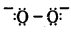all the electrons are paired and hence all peroxides are diamagnetic.

There are certain other oxides like PbCO2 and MnO2 which may be mistaken as peroxides. These compounds, however, do not give H2O2 on treatment with dilute acids. As such these compounds do not contain a peroxide ion $$\left(0_{2}^{2-}\right)$$ and hence they cannot be called as peroxides. Actually in these compounds the two oxygen atoms are linked to the metal atom by a double bond and hence called dioxide i.e, 0 = Pb = 0 (Lead dioxide) and O = Mn = O (mangenses dioxide). In dioxides, the oxidation state of each oxygen atom is -2.

b. Superoxides: Besides peroxides, alkali metals also form higher oxides called superoxides. For example, potassium superoxide (KO2), rubidium superoxide (RbO2) cesium superoxide (CSO2) etc. All these superoxides contain a superoxide ion, i.e., $$\mathrm{O}_{2}^{-}$$ having the structure,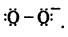Thus all superoxides contain an odd number of electrons (i.e. 13) and hence are paramagnetic.

1st PUC Chemistry The S-Block Elements Give Reasons

Question 1.
The ionic compounds of alkali metals are colourless, why?